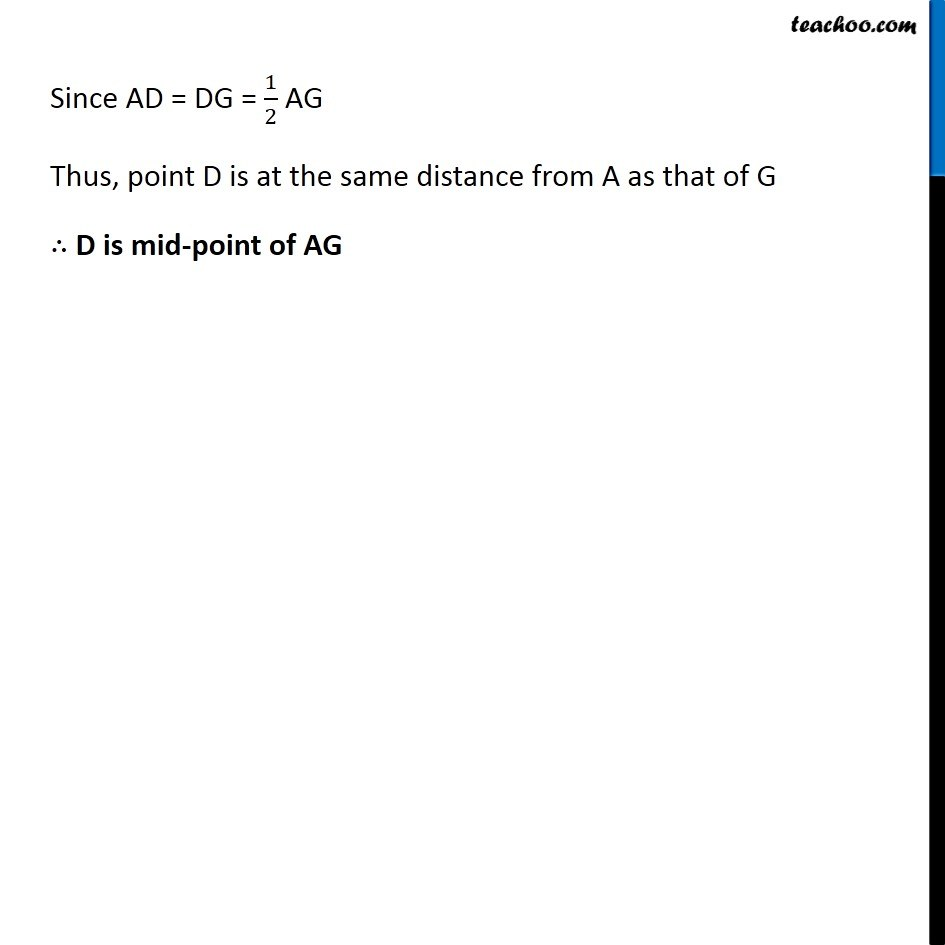Subscribe to our Youtube Channel - https://you.tube/teachoo

1. Chapter 5 Class 6 Understanding Elementary Shapes
2. Concept wise
3. Measuring Line Segments

Transcript

Ex 5.1, 5 Verify, whether D is the mid point of (𝐴𝐺) ̅ . We note that These are 6 blocks between A & G So , AG = 6 units And AD = 3 Units DG = 3 Units Since AD = DG = 1/2 AG Thus, point D is at the same distance from A as that of G ∴ D is mid-point of AG

Measuring Line Segments